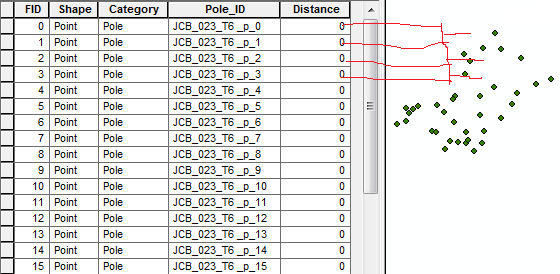# Calculate Distance.

4690
4
10-03-2015 05:06 AMby
New ContributorHello,

I need to update the distance field using the Pole_ID using Python script. From Pole_ID 0 to Pole_ID 1 and Pole_ID 1 to Pole_ID 2 and etc.

Kindly suggest me anyone.

4 RepliesbyMVP Esteemed Contributor

Inter-point distance

Do the following:

• select Python parser
• toogle on Show  code block
• paste the following in the Pre-logic Script Code block
• and the last piece in the expression box

Pre-logic Script Code:

``````""" input shape field: returns the distance between points and its center
dist_between(!Shape!)    #enter into the expression box"""
x0 = 0.0;  y0 = 0.0
def dist_between(shape):
global x0;  global y0
x = shape.firstpoint.X; y = shape.firstpoint.Y
if x0 == 0.0 and y0 == 0.0:
x0 = x; y0 = y
distance = math.sqrt((x - x0)**2 + (y - y0)**2)
x0 = x;  y0 = y
return distance
‍‍‍‍‍‍‍‍‍‍‍``````

calculation field =

``````dist_between(!Shape!)
‍``````

where calculation field is your active field, it will show its name.

Make sure you have projected data and a double field or nothing will make sense.byMVP Esteemed Contributor

Cumulative Distance

If you want distance from the first point then use cumulative distance:

repeat the steps from above

Pre-logic Script Code:

``````""" input shape field: returns cumulative distance between points
dist_cumu(!Shape!)    #enter into the expression box"""
x0 = 0.0;  y0 = 0.0;  distance = 0.0
def dist_cumu(shape):
global x0;  global y0;  global distance
x = shape.firstpoint.X;  y = shape.firstpoint.Y
if x0 == 0.0 and y0 == 0.0:
x0 = x; y0 = y
distance += math.sqrt((x - x0)**2 + (y - y0)**2)
x0 = x;  y0 = y
return distance
‍‍‍‍‍‍‍‍‍‍‍‍‍‍‍‍‍‍‍‍‍‍‍``````

calculation field =

``````dist_cumu(!Shape!)
‍‍‍``````

Same caveats as above.

NOTE:

if you have 3 or more points selected, then it will calculate the values with just the selection.byMVP Frequent Contributor

But, be aware that the attribute table is not guaranteed to be in PoleID order. Esp if you have done some editing.byMVP Esteemed Contributor

More calculations are shown here, if you didn't find the suggestions correct